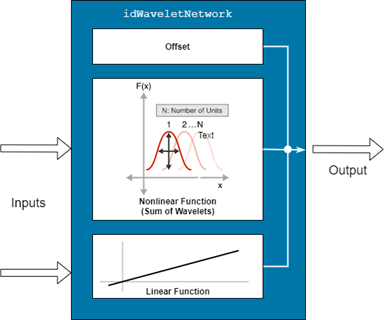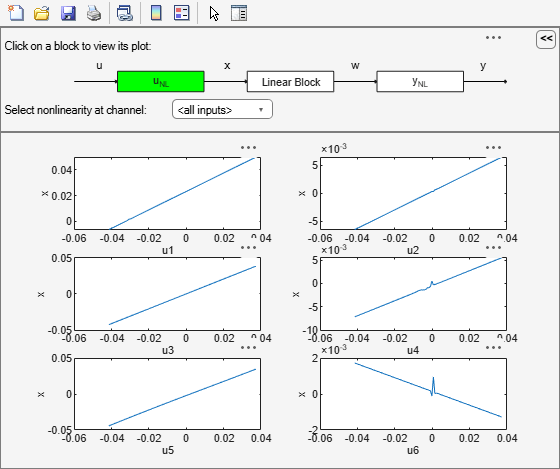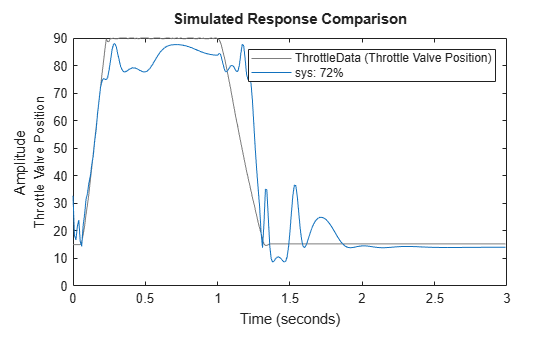# idWaveletNetwork

Wavelet network function for nonlinear ARX and Hammerstein-Wiener models

## Description

An `idWaveletNetwork` object implements a wavelet network function, and is a nonlinear mapping function for estimating nonlinear ARX and Nonlinear Hammerstein-Wiener models. The mapping function, which is also referred to as a nonlinearity, uses a combination of linear weights, an offset and a nonlinear function to compute its output. The nonlinear function contains wavelet unit functions that operate on a radial combination of inputs.Mathematically, `idWaveletNetwork` is a function that maps m inputs X(t) = [x(t1),x2(t),…,xm(t)]T to a scalar output y(t) using the following relationship:

`$y\left(t\right)={y}_{0}+{\left(Χ\left(t\right)-\overline{X}\right)}^{T}PL+W\left(Χ\left(t\right)\right)+S\left(Χ\left(t\right)\right)$`

Here:

• X(t) is an m-by-1 vector of inputs, or regressors, with mean $\overline{Χ}$.

• y0 is the output offset, a scalar.

• P is an m-by-p projection matrix, where m is the number of regressors and is p is the number of linear weights. m must be greater than or equal to p.

• L is a p-by-1 vector of weights.

• W(X) and S(X) together constitute the nonlinear function of the wavelet network. W(X) is a sum of dilated and translated wavelets while S(X) is a sum of dilated and translated scaling functions (also known as scalelets). The total number of wavelet dw and scaling functions ds is referred to as the number of units of the network.

For definitions of the wavelet function term W(X) and the scaling function term S(X), see More About.

Use `idWaveletNetwork` as the value of the `OutputFcn` property of an `idnlarx` model or the `InputNonlinearity` and `OutputLinearity` properties of an `idnlhw` object. For example, specify `idWaveletNetwork` when you estimate an `idnlarx` model with the following command.

`sys = nlarx(data,regressors,idWaveletNetwork)`
When `nlarx` estimates the model, it essentially estimates the parameters of the `idWaveletNetwork` function.

You can configure the `idWaveletNetwork` function to disable components and fix parameters. To omit the linear component, set `LinearFcn.Use` to `false`. To omit the offset, set `Offset.Use` to `false`. To specify known values for the linear function and the offset, set their `Value` attributes directly and set the corresponding `Free` attributes to `False`. Use `evaluate` to compute the output of the function for a given vector of inputs.

## Creation

### Syntax

``W = idWaveletNetwork``
``W = idWaveletNetwork(numUnits)``
``W = idWaveletNetwork(numUnits,UseLinearFcn)``
``W = idWaveletNetwork(numUnits,UseLinearFcn,UseOffset)``

### Description

example

````W = idWaveletNetwork` creates a `idWaveletNetwork` object `W`, for which the function computes the number of units automatically during model estimation. ```

example

````W = idWaveletNetwork(numUnits)` specifies the number of units `numUnits`. This syntax includes an option that allows you to interactively assess the relationship between the number of units and unexplained variance.```

example

````W = idWaveletNetwork(numUnits,UseLinearFcn)` specifies whether the function uses a linear function as a subcomponent.```

example

````W = idWaveletNetwork(numUnits,UseLinearFcn,UseOffset)` specifies whether the function uses an offset term.```

### Input Arguments

expand all

Number of units, specified as the string or character vector that represents `'auto'` or `'interactive'`, or as a positive integer. `numUnits` determines the number of wavelets or scaling functions, or, if both elements are present, the combined number of wavelets and scaling functions. Typically, the wavelet network contains either wavelets or scaling functions, but not both. Specify `numUnits` as one of the following values:

• `'auto'` — The software determines the number of units automatically during model estimation.

• `'interactive'` — During model estimation, the software displays an interactive bar plot that relates unexplained variance to the number of units. Click on a bar to view the achievable fit to the estimation data for the selected number of units. A blue bar indicates the optimal choice, based on the generalized cross-validation (GCV) criterion. A general rule for the selection of the number of units is to use the smallest number of units that capture most of the variance.

• Positive integer — The software uses the specified value directly.

This argument sets the `W.NonlinearFcn.NumberOfUnits` property.

Option to use the linear function subcomponent, specified as `true` or `false`. This argument sets the value of the `W.LinearFcn.Use` property.

Option to use an offset term, specified as `true` or `false`. This argument sets the value of the `W.Offset.Use` property.

## Properties

expand all

Input signal information for signals used for estimation, specified as vectors of m property-specific values, where m is the number of input signals. The `Input` properties for each input signal are as follows:

• `Name` — Names of the input signals, specified as a 1-by-m string or character array, where m is the number of inputs

• `Mean` — Mean of the input signals, specified as a numeric scalar

• `Range` — Ranges of the input signals, specified as a 2-by-m numeric array that contains the minimum and maximum values

Output signal information, specified as property-specific values. The `Output` properties are as follows:

• `Name` — Name of the output signal, specified as a string or a character array

• `Mean` — Mean of the output signal, specified as a numeric scalar

• `Range` — Range of the output signal, specified as a 2-by-1 numeric array that contains the minimum and maximum values

Parameters of the linear function, specified as follows:

• `Use` — Option to use the linear function in the wavelet network, specified as a scalar logical. The default value is `true`. For an example of setting this option, see Exclude Linear Term from Wavelet Network Mapping Object.

• `Value` — Linear weights that compose L', specified as a 1-by-p vector.

• `InputProjection` — Input projection matrix P, specified as an m-by-p matrix, that transforms the input vector of length m into a vector of length p. For Hammerstein-Wiener models, `InputProjection` is equal to `1`.

• `Free` — Option to update entries of `Value` during estimation, specified as a 1-by-p logical vector. The software honors the `Free` specification only if the starting value of `Value` is finite. The default value is `true`.

• `Minimum` — Minimum bound on `Value`, specified as a 1-p vector. If `Minimum` is specified with a finite value and the starting value of `Value` is finite, then the software enforces that minimum bound during model estimation.

• `Maximum` — Maximum bound on `Value`, specified as a 1-p vector. If `Maximum` is specified with a finite value and the starting value of `Value` is finite, then the software enforces that maximum bound during model estimation.

• `SelectedInputIndex` — Indices of `idWaveletNetwork` inputs (see `Input.Name`) that are used as inputs to the linear function, specified as an 1-by-nr integer vector, where nr is the number of inputs. For nonlinear ARX models, the `RegressorUsage` property determines these indices. For Hammerstein-Wiener models, `SelectedInputIndex` is always `1`.

Parameters of the offset term, specified as follows:

• `Use` — Option to use the offset in the wavelet network, specified as a scalar logical. The default value is `true`.

• `Value` — Offset value, specified as a scalar.

• `Free` — Option to update `Value` during estimation, specified as a scalar logical. The software honors the `Free` specification of `false` only if the value of `Value` is finite. The default value is `true`.

• `Minimum` — Minimum bound on `Value`, specified as a numeric scalar or `–Inf`. If `Minimum` is specified with a finite value and the value of `Value` is finite, then the software enforces that minimum bound during model estimation. The default value is `-Inf`.

• `Maximum` — Maximum bound on `Value`, specified as a numeric scalar or `Inf`. If `Maximum` is specified with a finite value and the starting value of `Value` is finite, then the software enforces that maximum bound during model estimation. The default value is `Inf`.

Parameters of the nonlinear function, specified as follows:

• `NumberOfUnits` — Number of units, specified as `'auto'`, `'interactive'`, or a positive integer. `NumberOfUnits` determines the number of wavelets or scaling functions, or, if both elements are present, the combined number of wavelets and scaling functions. Typically, the wavelet network contains either wavelets or scaling functions, but not both. The options for `NumberOfUnits` are as follows:

• `'auto'` — The software determines the number of units automatically during model estimation.

• `'interactive'` — During model estimation, the software displays an interactive bar plot that relates unexplained variance to the number of units. Click on a bar to view the achievable fit to the estimation data for the selected number of units. A blue bar indicates the optimal choice, based on the generalized cross-validation (GCV) criterion. A general rule for the selection of the number of units is to use the smallest number of units that capture most of the variance.

• Positive integer — The software uses the specified value directly.

• `Structure` — Advanced options that control the structure of the wavelet and scaling functions, specified as in the following table.

PropertyDescriptionDefault
`FinestCell`Minimum number of data points in the smallest cell, specified as `'auto'` or a positive integer. A cell is the area covered by the part of a wavelet that is significantly nonzero. The default setting of `'auto'` specifies that the software determines this value during estimation.`'auto'`
`MinimumCells`Minimum number of cells in the partition, specified as a positive integer.`16`
`MaximumCells`Maximum number of cells in the partition, specified as a positive integer.`16`
`MaximumLevels`Maximum number of wavelet levels, specified as a positive integer. `10`
`DilationStep`Dilation step size, specified as a positive integer.`2`
`TranslationStep`Translation step size, specified as a positive integer.`1`

• `Parameters` — Parameters of `idWaveletNetwork`, specified as in the following table.

Field NameDescriptionDefault
`InputProjection `

Projection matrix Q, specified as an m-by-q matrix. Q transforms the detrended input vector $\left(X-\overline{X}\right)$ of length m into a vector of length q. Typically, Q has the same dimensions as the linear projection matrix P. In this case, q is equal to p, which is the number of linear weights.

For Hammerstein-Wiener models, `InputProjection ` is equal to `1`.

`[]`
`ScalingCoefficient`

Scaling function coefficients si, specified as a ds-by-1 vector.

`[]`
`ScalingTranslation`

Scaling translation matrix, specified as a ds-by-q matrix of scaling translation row vectors ei.

`[]`
`ScalingDilation`

Scaling function dilation coefficients di, specified as an ds-by-1 vector.

`[]`
`WaveletCoefficient`

Wavelet function coefficients wi, specified as a dw-by-1 vector.

`[]`
`WaveletTranslation`

Wavelet translation matrix, ei, specified as a dw-by-q matrix of wavelet translation row vectors ci.

`[]`
`WaveletDilation`

Wavelet dilation coefficients bi, specified as an dw-by-1 vector.

`[]`

• `Free` — Option to estimate parameters, specified as a logical scalar. If all the parameters have finite values, such as when the `idWaveletNetwork` object corresponds to a previously estimated model, then setting `Free` to `false` causes the parameters of the nonlinear functions W(X) and S(X) to remain unchanged during estimation. The default value is `true`.

• `SelectedInputIndex` — Indices of `idWaveletNetwork` inputs (see `Input.Name`) that are used as inputs to the nonlinear function, specified as an 1-by-nr integer vector, where nr is the number of inputs. For nonlinear ARX models, the `RegressorUsage` property determines these indices. For Hammerstein-Wiener models, `SelectedInputIndex` is always `1`.

## Examples

collapse all

`MO = idWaveletNetwork;`

View the `idWaveletNetwork` object.

`disp(MO)`
```Wavelet Network Nonlinear Function: Wavelet network with number of units chosen automatically Linear Function: uninitialized Output Offset: uninitialized Input: '<Function inputs>' Output: '<Function output>' LinearFcn: '<Linear function parameters>' NonlinearFcn: '<Wavelet and scaling function units and their parameters>' Offset: '<Offset parameters>' EstimationOptions: '<Estimation options>' ```

Create the `idWaveletNetwork` mapping object `MO`.

`MO = idWaveletNetwork;`

Exclude the linear term from `MO`.

`MO.LinearFcn.Use = false;`

View the `idWaveletNetwork` object.

`disp(MO)`
```Wavelet Network Nonlinear Function: Wavelet network with number of units chosen automatically Linear Function: not in use Output Offset: uninitialized Input: '<Function inputs>' Output: '<Function output>' LinearFcn: '<Linear function parameters>' NonlinearFcn: '<Wavelet and scaling function units and their parameters>' Offset: '<Offset parameters>' EstimationOptions: '<Estimation options>' ```

The linear function is not in use.

`load twotankdata y u;`

Create an `iddata` object from the estimation data.

`z = iddata(y,u,0.2);`

Create a wavelet network mapping object with five units.

`MO = idWaveletNetwork(5);`

Estimate the nonlinear ARX model.

`sys = nlarx(z,[4 4 1],MO)`
```sys = Nonlinear ARX model with 1 output and 1 input Inputs: u1 Outputs: y1 Regressors: Linear regressors in variables y1, u1 List of all regressors Output function: Wavelet network with 5 units Sample time: 0.2 seconds Status: Estimated using NLARX on time domain data "z". Fit to estimation data: 96.8% (prediction focus) FPE: 3.553e-05, MSE: 3.515e-05 ```

`load motorizedcamera;`

Create an `iddata` object.

`z = iddata(y,u,0.02,'Name','Motorized Camera','TimeUnit','s');`

`z` is an `iddata` object with six inputs and two outputs.

Specify the model orders and delays.

`Orders = [ones(2,6),ones(2,6),ones(2,6)];`

Specify the same nonlinearity estimator for each input channel.

`InputNL = idWaveletNetwork;`

Specify different nonlinearity estimators for each output channel.

` OutputNL = [idDeadZone,idWaveletNetwork];`

Estimate the Hammerstein-Wiener model.

`sys = nlhw(z,Orders,InputNL,OutputNL);`

To see the shape of the estimated input and output nonlinearities, plot the nonlinearities.

`plot(sys)`Click on the input and output nonlinearity blocks on the top of the plot to see the nonlinearities.

`load iddata7 z7;`

Specify `idWaveletNetwork` for the model output function. Configure the `idWaveletNetwork` object to have a fixed offset value of 1 and to use five units in the nonlinear component.

```w = idWaveletNetwork; w.Offset.Value = 1; w.Offset.Free = false; w.NonlinearFcn.NumberOfUnits = 5;```

Specify a linear model regressor set using a lag array that produces four consecutive output regressors and five consecutive input regressors.

`reg = linearRegressor({'y1','u1'},{1:4,0:4});`

Estimate the nonlinear ARX model.

`sys = nlarx(z7,reg,w);`

Examine the postestimation properties of the output function.

`disp(sys.OutputFcn)`
```Wavelet Network Inputs: y1(t-1), y1(t-2), y1(t-3), y1(t-4), u1(t), u1(t-1), u1(t-2), u1(t-3), u1(t-4) Output: y1 Nonlinear Function: Wavelet network with 5 units Linear Function: initialized to [-1.12 0.469 1.25 0.556 -0.81 -0.261 -0.074 0.711 1.15] Output Offset: fixed to 1 Input: '<Function inputs>' Output: '<Function output>' LinearFcn: '<Linear function parameters>' NonlinearFcn: '<Wavelet and scaling function units and their parameters>' Offset: '<Offset parameters>' EstimationOptions: '<Estimation options>' ```
`disp(sys.OutputFcn.Input)`
```Function inputs Name: {1x9 cell} Mean: [0.0795 0.0848 0.1082 0.1134 0.0253 0.0253 0.0202 0.0202 0.0202] Range: [2x9 double] ```
`disp(sys.OutputFcn.NonlinearFcn)`
```Wavelet and scaling function units and their parameters NumberOfUnits: 5 Parameters: '<Wavelet parameters>' Free: 1 SelectedInputIndex: [1 2 3 4 5 6 7 8 9] ```

`load throttledata`

Specify `idWaveletNetwork` for the model output nonlinearity and set the arguments for `NumUnits` to `5` and `UseLinearFcn` and `UseOffset` to `false`.

`w = idWaveletNetwork(5,false,false);`

An alternative method for removing the linear function and offset is to use dot notation after creating the nonlinearity.

```w.LinearFcn.Use = false; w.Offset.Use = false;```

Estimate the model, using an `order` specification of `[4 4 1]`.

`sys = nlhw(ThrottleData,[4 4 1],[],w);`

Compare the simulated model response with the estimation data.

`compare(ThrottleData,sys);`expand all

## Algorithms

You can use `idWaveletNetwork` in both nonlinear ARX and Hammerstein-Wiener models. The algorithms for estimating `idWaveletNetwork` parameters depend on which model you are estimating.

• In a nonlinear ARX model, `idWaveletNetwork` uses either a noniterative or an iterative technique for predicting the parameters, depending on option settings in `nlarxOptions`.

• If the `Focus` option is set to `'prediction'`, then `idWaveletNetwork` uses a fast noniterative technique to estimate parameters . Successive refinements after the first estimation use an iterative algorithm.

• If the `Focus` option is set to `'simulation'`, then `idWaveletNetwork` uses an iterative technique to estimate parameters.

• To always use either an iterative or a noniterative algorithm, specify the `IterativeWavenet` property of `nlarxOptions` as `'on'` or `'off'`, respectively.

• In a Hammerstein-Wiener model, `idWaveletNetwork` uses iterative minimization to determine the parameters.

## Compatibility Considerations

expand all

Not recommended starting in R2021b

Not recommended starting in R2021a

 Qinghua Zhang. “Using Wavelet Network in Nonparametric Estimation.” IEEE Transactions on Neural Networks 8, no. 2 (March 1997): 227–36. https://doi.org/10.1109/72.557660.

## SupportGet trial now